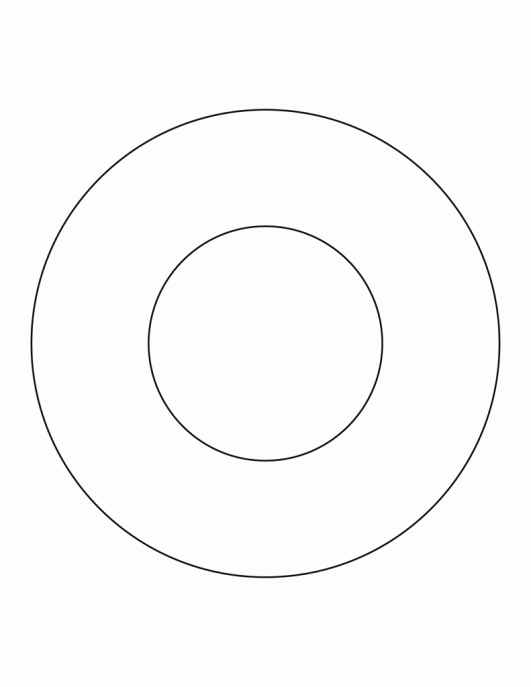# A sidewalk that is 3 ft wide surrounds a circular garden with a radius of 10 ft. What is the area of the side walk? use pi=3.14

I believe the area of the sidewalk is 216.66 sq ft.

Here's how I worked out the problem:

I started off by trying to find the respective areas of two different circles, one within the other. This sounds difficult, but is quite straightforward if you break down the task into separate parts.First, let's ignore the inner circle (the garden) and focus on the total area the 'outer' circle covers in total, in other words the area of the space covered by the path and garden combined.

We can do this as we have been given two important measurements, the radius of the garden (10 ft) and the width of the pathway (3 ft). If we lined up these two measurements, they would in effect gives us the radius of the total circular area, 13 ft. (This also means the diameter would be 26 ft, but we don't need that right now).

The Area of a Circle is equal to Radius Cubed x Pi

The cube of 13 is 13 x 13 = 169.

Pi = 3.14, so the area of the overall circle is 3.14 x 169 = 530.66 sq ft.

This gives us the amount in sq ft of the total area in the question, but we need to know the area of the pathway surrounding the smaller inner circle, the garden. So, all we need to do is subtract the area covered by the garden and pathway combined (530.66 sq ft) by the area covered by the garden. We already know the radius of the garden is 10 sq ft, so:

A = Pi x radius cubed:

3.14 x (10 x 10) = 314 sq feet. The area of the garden is 314 sq ft.

The remainder of the total area is the area covered by the path.

530.66 sq ft - 314 sq ft = 216.66 sq ft.

Here's more on finding out the area of circles:

thanked the writer.